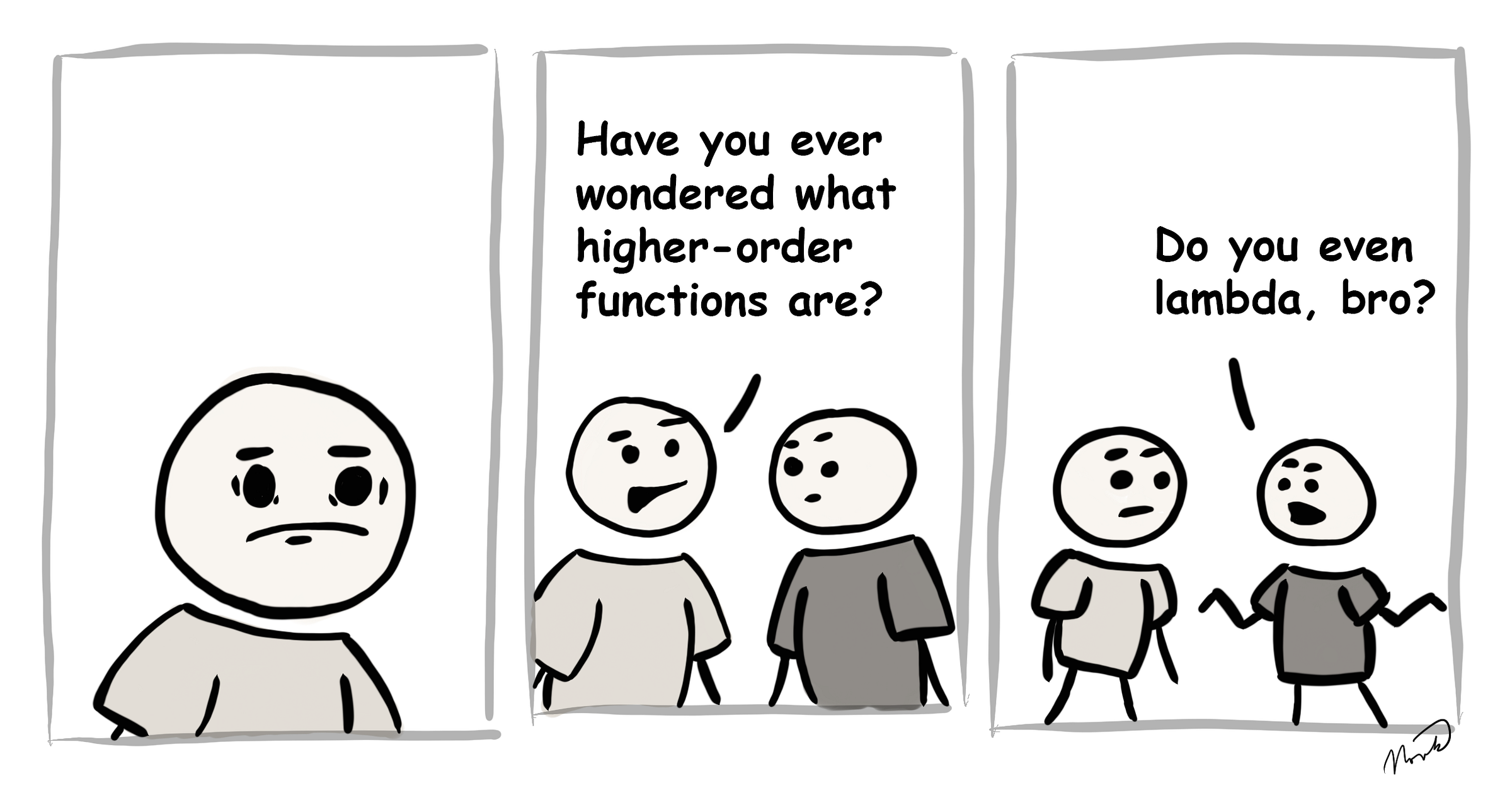# 6 fundamental terms in functional JavaScriptDo you even lambda, bro?

Let’s talk about what are lambdas, first-class functions, higher-order functions, unary functions, currying and pure functions.

If you are not clear on the difference between imperative and declarative programming, please consider reading my post: Imperative versus declarative code… what’s the difference?

### What is a lambda => arrow function?

Lambdas (λ) are better known in JavaScript as arrow functions:

`// this is your regular named function in JavaScriptfunction namedFunction (a, b) {  return a + b;}`
`// this is a lambda, i.e. an arrow functionconst lambda = (a, b) => a + b;`

The term lambda originates from lambda calculus, a formal system of mathematical logic. Lambda calculus is of course Turing complete and as such it represents a universal model of computation able to build any Turing machine.

Lambda expressions are the cornerstone of functional programming. If it helps you, just think of them as a new shorter syntax for your functions. However, be careful about using them in your objects or classes as they use lexical `this`.

### What is a first-class function?

First-class type means simply, that the type can be used as a value of a variable. A string is a first-class type as well as a function is a first-class type in JavaScript and so your functions can accept other functions as their arguments and return functions as their return values.

You have already been using functions as first class when assigning handlers to listeners:

`const handler = () => console.log ('I am function');`
`document.addEventListener ('click', handler); `

### What is a higher-order function?

Higher-order function is a function that accepts other function as an argument or returns a function as a return value.

First-order function is a function that doesn’t accept other function as an argument and doesn’t return a function as its return value.

`const firstOrder = () => console.log ('First order strikes back!');`
`const higherOrder = whoStrikesBack => whoStrikesBack ();`
`higherOrder (firstOrder);`

### What is a unary function?

The term relates to arity of a number of arguments accepted by a function. Unary function (i.e. monadic) is a function that accepts exactly one argument.

`const unaryFunction = message => console.log (message);`
`const binaryFunction = (color, message) =>  console.log (`%c\${message}`, `color:\${color}`);`
`const ternaryFunction = (fnc, color, message) =>  fnc (`%c\${message}`, `color:\${color}`);`

### What is currying?

Currying is the process of taking a function with multiple arguments and turning it into a sequence of functions each with only a single argument. Therefor with currying you take a n-ary function and you turn it into a unary function:

`const binaryFunction = (a, b) => a + b;`
`const curryUnaryFunction = a => b => a + b;`
`curryUnaryFunction (1); // returns a function: b => 1 + b`
`curryUnaryFunction (1) (2); // returns the number 3`

Currying is named after a mathematician Haskell Curry, not the food.

Curried functions are great to improve code reusability and functional composition. If you want to know more, read my post: JavaScript ES6 curry functions with practical examples. It may get some getting used to, but all functions, that I write nowadays, are unary functions thanks to currying.

### What is a pure function?

A pure function is a function where the return value is only determined by its arguments without any side effects.

That means that if you give a pure function the same argument a hundred times in a hundred different places of your whole application, the function will always return the same value. No external states will be changed or read by the pure function.

`let myArray = [];`
`const impureAddNumber = number => myArray.push (number);`
`const pureAddNumber = number => anArray =>  anArray.concat ([number]);`
`console.log (impureAddNumber (2)); // returns 1console.log (myArray); // returns console.log (pureAddNumber (3) (myArray)); // returns [2, 3]console.log (myArray); // returns myArray = pureAddNumber (3) (myArray);console.log (myArray); // returns [2, 3]`

`Push` function is impure itself and it alters the array it is called on and as such produces a side effect. The return value of push is a number index.

`Concat` on the other hand takes the array and concatenates it with the other array producing a whole new array without side effects. The new array as concatenation of the previous array is then returned.

Pure functions are important as they simplify unit testing (no side effects and no need for dependency injection), they avoid tight coupling and by removing side effects, they make your application harder to break.

I dedicated a full article to discussing how pure functions relate to best practices in programming: JavaScript Pure Functions for OOP developers.

### Conclusion

Understanding functional programming doesn’t make you just a better developer, it makes you a better person. You get to entertain your friends over a beer with lambda calculus and you can correct your family by fun implications of mathematical logic.

To use all of these terms in practice, continue by reading: 8 steps to turn imperative JavaScript class to a functional declarative code.

Also, to learn more about conditional statements and conditional expressions follow up with my post: How to replace switch and ternaries in functional JavaScript.